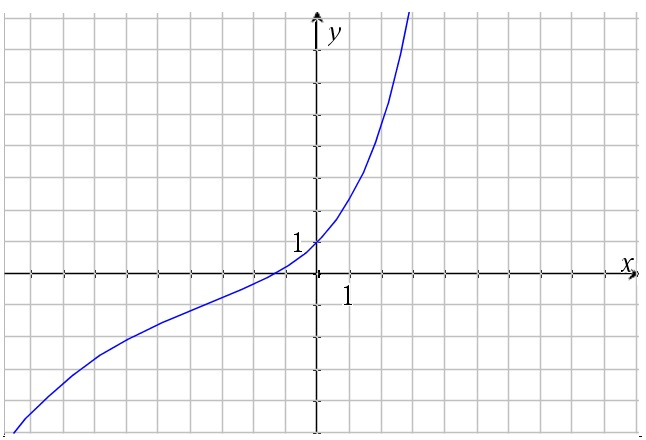# Some interesting examples based on the Fibonacci series

Up a level : Power Series
Previous page : Series - some general rules
Next page : Proof that ratios of Fibonacci successive numbers tend to the Golden ratioLet Fn be the nth Fibonacci number where F1 = F2 =1,  and Fn+1 = Fn+ Fn-1.

Let us create some functions${f_1}(x) = \sum\limits_{k = 0}^\infty {{F_{k + 1}}{x^k}}$${f_2}(x) = \sum\limits_{k = 0}^\infty {\frac{1}{{{F_{k + 1}}}}{x^k}}$${f_3}(x) = \sum\limits_{k = 0}^\infty {\frac{{{F_{k + 1}}}}{{k!}}{x^k}}$${f_4}(x) = \sum\limits_{k = 0}^\infty {\frac{1}{{k!{F_{k + 1}}}}{x^k}}$

Before you read any further, I suggest you try to find the radius of convergence for each of them. One little useful fact though,$\mathop {\lim }\limits_{k \to \infty } \frac{{\;{F_{k + 1}}\;}}{{{F_k}}} = \frac{{1 + \sqrt 5 }}{2}$

i.e. the golden ratio. We will prove that in the next page, but you might give it a go first.

The first function${f_1}(x) = \sum\limits_{k = 0}^\infty {{F_{k + 1}}{x^k}}$

We have$\left| {{u_k}} \right| = \left| {{F_{k + 1}}{x^k}} \right|$

and thus$\begin{gathered}\mathop {\lim }\limits_{k \to 0} \left| {\frac{{{u_{k + 1}}}}{{{u_k}}}} \right| = \mathop {\lim }\limits_{k \to 0} \left| {\frac{{\;{F_{k + 2}}{x^{k + 1}}\;}}{{{F_{k + 1}}{x^k}}}} \right| \hfill \\ \quad = \mathop {\lim }\limits_{k \to 0} \left| {\frac{{\;{F_{k + 2}}\;}}{{{F_{k + 1}}}} \cdot x} \right| = \frac{{1 + \sqrt 5 }}{2}\left| x \right| > 1 \hfill \\ \end{gathered}$

So$\frac{{1 + \sqrt 5 }}{2}\left| x \right| < \frac{2}{{1 + \sqrt 5 }} = \frac{{\sqrt 5 - 1}}{2}$

That would be the radius of convergence. To find the interval is another matter, but let us concentrate on the radius of convergence.

The second function${f_2}(x) = \sum\limits_{k = 0}^\infty {\frac{1}{{{F_{k + 1}}}}{x^k}}$

Hopefully quite obviously this function will have the radius of convergence of$\frac{{1 + \sqrt 5 }}{2}$

The two series of the two functions are plotted below. Both using 150 terms. The dotted lines correspond to the radius of convergence for respective series. The blue graph correspond to the first function and the red line to the second. A couple of questions arises from the graphs. What is the value the first graph is approaching as it is approaching the lower end of the interval of convergence. It seems to be just slightly larger than 0.8. What is the minimum point of the graph? It seems to be 0.8.Is the second function actually negative at the lower end of its interval of convergence? Also, is the first function larger than the second over its whole range? These are questions to examine at some other time.

The third function${f_3}(x) = \sum\limits_{k = 0}^\infty {\frac{{{F_{k + 1}}}}{{k!}}{x^k}}$$\left| {{u_k}} \right| = \left| {\frac{{{F_{k + 1}}}}{{k!}}{x^k}} \right|$

This gives us$\begin{gathered} \mathop {\lim }\limits_{k \to 0} \left| {\frac{{{u_{k + 1}}}}{{{u_k}}}} \right| = \mathop {\lim }\limits_{k \to 0} \left| {\frac{{\;\frac{{{F_{k + 2}}{x^{k + 1}}}}{{(k + 1)!}}\;}}{{\frac{{{F_{k + 1}}{x^k}}}{{k!}}}}} \right| \hfill \\ \quad = \mathop {\lim }\limits_{k \to 0} \left| {\frac{{{F_{k + 2}}}}{{(k + 1)!}} \cdot \frac{{k!}}{{{F_{k + 1}}}} \cdot x} \right| = \mathop {\lim }\limits_{k \to 0} \left| {\frac{{{F_{k + 2}}}}{{{F_{k + 1}}}} \cdot \frac{1}{{k + 1}} \cdot x} \right| \hfill \\ \quad = \mathop {\lim }\limits_{k \to 0} \left| {\frac{{{F_{k + 2}}}}{{{F_{k + 1}}}}} \right| \cdot \mathop {\lim }\limits_{k \to 0} \left| {\frac{{x1}}{{k + 1}}} \right| = 0 \hfill \\ \end{gathered}$

This will thus converge for any value of x. Yet again there are some questions. When x>0 it seems to grow approximately as 5.012x and when x<-1 as 0.776.0·0.537x. I found that by graphing the log base 10 of the function, as seen in the figure below.The strange knee to the left seems to be an artefact of the limited number of terms, as it will move to the left as more terms are added.

The fourth function

It should be fairly easy to see that this is also convergent for all values of x. A graph using the first 40 terms is shown below.Up a level : Power Series
Previous page : Series - some general rules
Next page : Proof that ratios of Fibonacci successive numbers tend to the Golden ratioLast modified: Mar 10, 2019 @ 20:30